Study Guide

# Variations

## Variations

Ever been to a themed party? Perhaps "Under the Sea" strikes your fancy. Imagine all the possibilities. You and your buddies could dress as sharks, clownfish, or manatees. Or maybe fictitious animals are more your thing? You could hit the party as a mermaid, the Loch Ness Monster, or Ursula (the sea witch).

Math can also have variations on a theme. Two variables (usually x and y) can have all kinds of different relationships in an equation. The most basic type is direct variation. Others include inverse variation, joint variation, and combined variation. Like we said, lots of variations.

• ### Direct Variation

As the song sort of goes, what goes up goes up, and what goes down goes down.

The general form for direct variation looks like this:

y = kx

This states that y varies directly with x, where k is a constant of variation. All that really means is that when x increases, y also increases. And as x decreases, y decreases.

Another way to say this is y is directly proportional to x. Or that y is in direct proportion to x.

Let's look an example of direct variation. If Marge is paid \$8 an hour for babysitting eight pit bulls, then the amount per job depends on the number of hours she watches the snarling pups. We can turn this into an equation:

y = 8x

...where x represents the number of hours she worked and y represents how much money she'll make.

### Sample Problem

A case of Red Bull Super X energy drinks has 24 bottles. How many bottles do 10 cases hold?

Let y be the number of bottles and x be the number of cases. The number of bottles varies directly with the number of cases.

Each case holds 24 bottles, so our equation is:

y = 24x

That means k, our constant of variation, is 24.

For our problem, x = 10 cases. Plugging that in gives us:

y = (24)(10)

Which finally gives us:

y = 240 bottles

### Sample Problem

If y varies directly with x, and y = 20 when x = 4, find y when x = 10.

First, write the general form for direct variation:

y = kx

Plug in the given values for x and y, and solve for k.

20 = k(4)

k = 5

Now, plug k = 5 back into the general form

y = 5x

We can now find y when x = 10.

y = 5(10)

y = 50

### Sample Problem

The circumference of a circle (say, a circular firing squad) varies directly with its diameter. Write the equation to represent this variation and find the constant of variation.

Let y represent the circumference of the circle (er, squad) and x be the diameter.

y = kx

Comparing this to the known formula for the circumference of the circle C = πd shows us that in this case:

k = π

• ### Inverse Variation

If you thought direct variation was a cinch, welcome to inverse variation. When two variables vary inversely, that means what goes up goes down, and what does down goes up. This numerical seesawing goes on and on till you get dizzy or solve the problem—whatever comes first.

In algebra-land, here's the general form for inverse variation: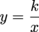This states y varies inversely with x, where k is still a constant of variation. "Inversely" means that as x increases, y decreases, and as x decreases, y increases.

Another way to say this is y is inversely proportional to x. Or that y is in inverse proportion to x.

We can also rearrange that inverse variation formula as k = xy. Notice that when x increases, y decreases so that the product k remains the same.

Still having fun, inversions and all? Check out the sample problems below.

### Sample Problem

If y varies inversely with x, and y = 8 when x = 2, find y when x = 4.

First, write the general form for inverse variation.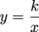Now plug in our values for x and y to find k.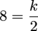Solve for k.

k = 16

Plug k back into the general form for inverse variation.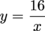To finish up, we find y when x = 4.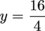Which means y = 4.

### Sample Problem

If y is inversely proportional to x2, and y = 4 when x = 5, find y when x = 10.

Write the general form for inverse variation. This time, we're told y varies inversely with x2, not just plain-old x.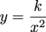Now plug in our values for x and y to find k.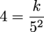Solve for k.

k = 100

Plug k back into the general form for inverse variation.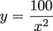Now find y when x = 10.### Sample Problem

For gases at a constant temperature—sewer gas, for example—volume varies inversely with pressure. If a gas has a volume of 50.0 L at a pressure of 2.00 atm, find its volume at a pressure of 4.00 atm.

First, write the general form for inverse variation.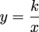Let's replace y with a V for volume and x with P for pressure.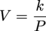Now plug in our values for V and P to find k.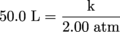Solve for k.

k = 100

Plug k back into the general form for inverse variation.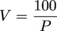Now find V when x = 4.00.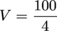V = 25.0 L

That's still pretty gassy. Does it reek in here, or is it just us?

• ### Joint Variation

Now, let's deal with joint variation, where one is never enough. In fact, one seriously depends on two others, making a mathematical trio. Turns out it's not so fancy-shmancy: joint variation is like direct variation, but it involves more than one variable. The general form looks like this:

y = kxz

...where y varies directly with x, and y also varies directly with z. In other words, y varies directly with the product of xz. The constant of variation is still our good buddy, k.

Typically, we use this formula to find the area of a rectangle:

A = lw

Here k = 1.

If either the length or the width increases, the area increases. If either the length or the width decreases, the area decreases. Just think of lots of moving parts, geometrically speaking.

### Sample Problem

If y varies jointly with the product of x and z, and y = 72 when x = 4 and z = 9, find y when x = 5 and z = 6.

First, we bust out our general form for joint variation.

y = kxz

Plug in our values to find k.

72 = k(4)(9)

Solve for k.

72 = 36k

k = 2

Plug k back into our general form.

y = 2xz

Finally, find y when x = 5 and z = 6.

y = 2(5)(6)

y = 60

### Sample Problem

If y varies jointly with the product of x and z, and y = 40 when x = 1 and z = 2, find y when x = 3 and z = 4.

y = kxz

Plug in everything we know.

40 = k(1)(2)

Now we can find k.

40 = 2k

k = 20

Throw k back into our general equation.

y = 20xz

Last but definitely not least, find y when x = 3 and z = 4.

y = 20(3)(4)

y = 240

### Sample Problem

If y varies jointly with the product of x and z, and y = 30 when x = 10 and z = 6, find y when x = 7 and z = 2.

Not to sound like a broken record here, but once again, we whip out the general formula.

y = kxz

Throw in our values for x, y, and z, then stir the pot and heat at 350°.

30 = k(10)(6)

Solve for k.

30 = 60k

k = 0.5

Plug k back into our general form.

y = 0.5xz

Demolish this problem by finding y when x = 7 and z = 2.

y = 0.5(7)(2)

y = 7

• ### Combined Variation

Last, but in no way least, is combined variation. Numbers are mixed, matched, computed, jumbled, and ultimately combined.

Combined variation mixes both direct and inverse variation. The general form looks like this: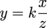Here, y varies directly with x, and y varies inversely with z. It's a double dose of variation. If x increases, y increases. If x decreases, y decreases. If z increases, y decreases. If z decreases, y increases.

Confused yet? Naw, take it one step at a time. Check out the sample problems below.

### Sample Problem

If y varies directly with x and inversely with z, and y = 36 when x = 12 and z = 2, find y when x = 6 and z = 3.

First, write the general form for combined variation.Plop in those given values and solve for k.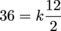36 = 6k

k = 6

Now, plug our k-value into the general equation.All that's left is to find y when x = 6 and z = 3.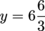y = 6(2) = 12

Owned.

### Sample Problem

If y varies directly with x and inversely with z, and y = 5 when x = 100 and z = 5, find y when x = 8 and z = 4.

Where's our combined variation formula? We know we stuck it around here somewhere...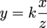Ah, there it is. Plug in everything we know and solve for k.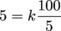5 = 20k

k = 0.25

That gives us our general equation.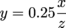Now, find y when x = 8 and z = 4.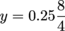y = 0.25(2) = 0.5

### Sample Problem

If y varies directly with x and inversely with z, and y = 20 when x = 10 and z = 6, find y when x = 15 and z = 3.

We can do this in our sleep by now. Kick things off with the general formula.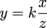Plug in the given values and solve for k.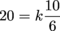Multiply both sides by 6 to drop that fraction.

120 = 10k

k = 12

Throw that brand-spanking-new k into the general equation.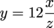Hunt down y when x = 15 and z = 3.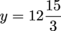y = 12(5) = 60

That's all, folks. If the world ever seems wacky and out of control, you can always fiddle around with math and find refuge in rational functions.